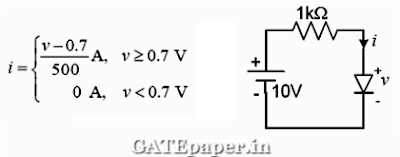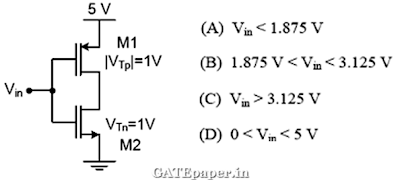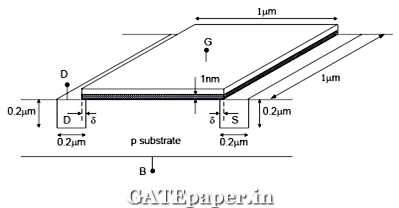### GATE 2012 ECE Video Solutions on EDC (Electronic Devices and Circuits)

1. The I – V characteristics of the diode in the circuit is given below, then the current in the circuit isa. 10 mA
b. 9.3 mA
c. 6.67 mA
d. 6.2 mA

2. The diodes and capacitors shown in the circuit are ideal. The voltage V(t) across the diode D1 is3. In the circuit shown below, the output expression ‘Y’ is4. In the CMOS circuit shown, electron and hole motilities are equal, and M1 and M2 transistors are equally sized. The device M1 is in the linear region if5. In the three dimensional view of a silicon N channel MOS transistor shown below, δ = 20 nm. The transistor is of width 1 µm. The depletion width formed at every PN junction is 10 nm. The relative permittivity’s of Si and SiO2 are 11.7 and 3.9 respectively and Ɛ0 = 8.9 X 10-12 F/m.i. The gate – source overlap capacitance is approximately
a. 0.7 fF
b. 0.7 pF
c. 0.35 fF
d. 0.24 fF

ii. The source – body capacitance approximately
a. 2 fF
b. 7 fF
c. 2 pF
d. 7 pF